• 矩形面积交 （C语言代码）
2021-05-18 16:48:45

解题思路:

注意事项:

参考代码:#include

int main(){

double a1,a2,b1,b2,c1,c2,d1,d2,e1,e2,f1,f2;

scanf("%lf %lf %lf %lf",&a1,&a2,&b1,&b2);

scanf("%lf %lf %lf %lf",&c1,&c2,&d1,&d2);

//求出两矩形左下和右上两个点坐标

if(a2>b2){double t=b2;b2=a2;a2=t;}

if(c2>d2){double t=d2;d2=c2;c2=t;}

if(a1>b1){double t=b1;b1=a1;a1=t;}

if(c1>d1){double t=d1;d1=c1;c1=t;}

//无交集

if(a1>=d1||a2>=d2||b1<=c1||b2<=c2)printf("0.00");

//求重合矩形的左下和右上两点坐标

else{

e1=a1>=c1?a1:c1;

e2=a2>=c2?a2:c2;

f1=b1<=d1?b1:d1;

f2=b2<=d2?b2:d2;

printf("%.2f",(f1-e1)*(f2-e2));

}

}

更多相关内容
• 其信息包括：矩形中心点 row,column矩形角度 Phi矩形区域的长和宽的一半 Length1, Length2如下图所示：(图1)就没有没直接给出矩形4个顶点坐标。有时候想知道4个顶点的坐标怎么计算呢？由于勇哥手上...

已经矩形的中心点、边长、phi求四个顶点的坐标

在halcon里面，类似gen_measure_rectangle2、smallest_rectangle2的算子表示任意角度的矩形。

其信息包括：矩形中心点 row,column

矩形角度 Phi

矩形区域的长和宽的一半 Length1, Length2

如下图所示：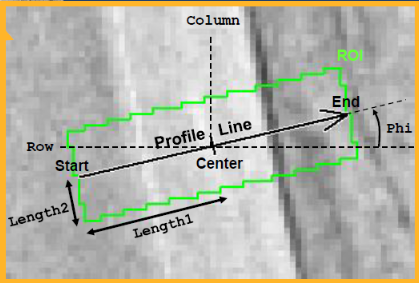(图1)

就没有没直接给出矩形4个顶点坐标。有时候想知道4个顶点的坐标怎么计算呢？

由于勇哥手上有一段halcon代码正好用解决上面的问题，所以我们先分析一下代码的结果，以此为参考进行推导。

代码如下：* Image Acquisition 01: Code generated by Image Acquisition 01

gen_rectangle1 (ROI_0, 703.23, 1022.93, 2188.17, 3252.27)

reduce_domain(Image, ROI_0, ImageReduced)

threshold(ImageReduced, Region, 0, 100)

connection(Region, ConnectedRegions)

select_shape(ConnectedRegions, SelectedRegions, 'area', 'and', 15000, 999999999)

smallest_rectangle2 (SelectedRegions, Row16, Column16, Phi3, Length1, Length2)

tuple_deg(Phi3,Deg)

len1:=Column16-Length1

len2:=Row16-Length2

*提取仿射最小外接矩形的四个顶点坐标

gen_rectangle2_contour_xld (Rectangle4, Row16, Column16, Phi3, Length1, Length2)

tuple_cos (Phi3, Cos)

tuple_sin (Phi3, Sin)

dev_set_color('red')

a:= -Length1*Cos - Length2*Sin

b := -Length1*Sin + Length2*Cos

gen_cross_contour_xld(Cross1, Row16-b,  Column16+a, 6, Phi3)

c := Length1*Cos - Length2*Sin

d := Length1*Sin + Length2*Cos

gen_cross_contour_xld(Cross2, Row16-d, Column16+c, 6, Phi3)

e:= Length1*Cos + Length2*Sin

f := Length1*Sin - Length2*Cos

gen_cross_contour_xld(Cross3, Row16-f, Column16+e, 6, Phi3)

g := -Length1*Cos + Length2*Sin

h := -Length1*Sin - Length2*Cos

dev_set_color('green')

x1:=Column16+a

y1:=Row16-b

disp_obj(Cross1,200000)

在下图中，矩形的四个顶点坐标位置被显示了出来。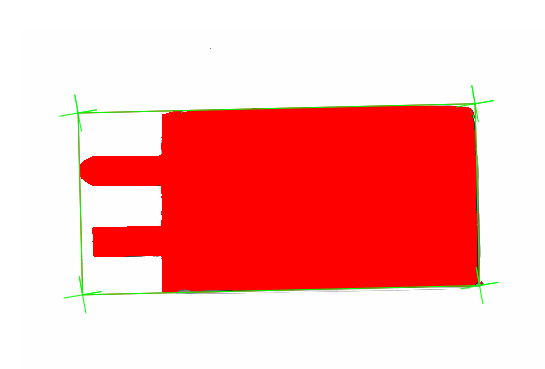(图2)

从上面的halcon代码结果中，我们可以观察到矩形左上角顶点的数据如下：Length1=807.053,  Length2=378.839

Phi3=0.0239714弧度=1.37346度

矩形中心坐标：x=2067.95  y=1413.22

cos(1.37346)=0.9997127

sin(1.37346)=0.0239691034

a:= -Length1*Cos - Length2*Sin

b:= -Length1*Sin + Length2*Cos

a=-815.902

b=359.386

矩形左顶点坐标：

x=2067.95+a=1252.04843

y=1413.22-b=1053.83418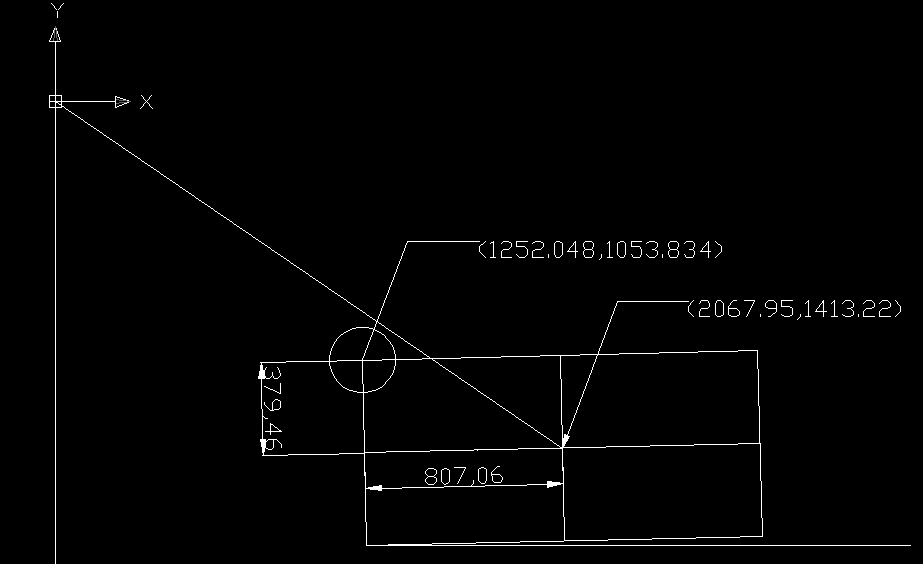(图3)

0.9997127

命令: sin(1.37346)

未知命令“SIN(1.37346)”。按 F1 查看帮助。

命令: cal

>> 表达式: sin(1.37346)

0.0239691034

命令: cal

>> 表达式: -807.053*cos(1.37346)-378.839*sin(1.37346)

-815.901565

命令: cal

>> 表达式: -807.053*sin(1.37346)+378.839*cos(1.37346)

359.385823

命令: CAL

>> 表达式: 2067.95-815.901565

1252.04843

命令: cal

>> 表达式: 1413.22-359.385823

1053.83418

对于代码中的计算公式矩形中心坐标：x=2067.95  y= 1413.22

a:= -Length1*Cos - Length2*Sin=-815.902

b:= -Length1*Sin + Length2*Cos=359.386

x0=x+a=1252.04843

y0=y-b=1053.83418

可能仍然不好理解，其实它是上一篇《三角函数在图形学里的应用(二)》中所说的饶任意点旋转公式的应用。

这里再引用一下那个公式：x0=cos(a)*(x-rx0)-sin(a)*(y-ry0)+rx0

y0=cos(a)*(y-ry0)+sin(a)*(x-rx0)+ry0

其中x,y为起始点，x0,y0是旋转后的点，rx0,ry0是旋转中心, a是旋转的角度

在下面的示意图中，白色矩形是水平状态的矩形，黄色矩形是转了a度的矩形。

图中标注出了矩形的中心坐标、旋转前的左上角坐标、旋转后的左上角坐标。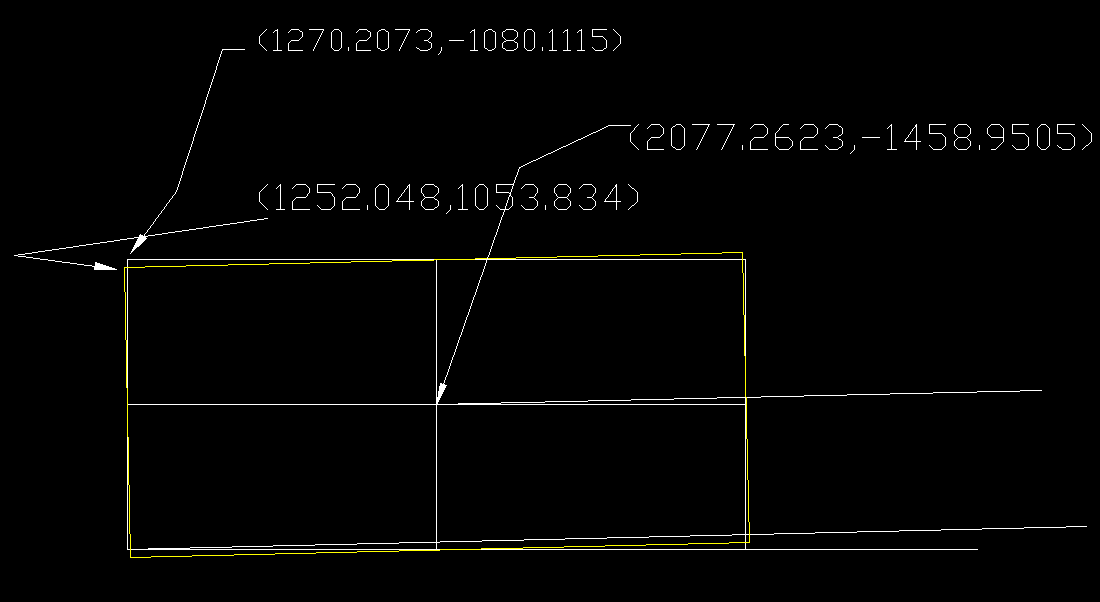(图4)

按图中的信息，勇哥用cad计算了验证了一下，可以看到结果完全正确：rx0 = 2077.2623，  ry0 = -1458.9505    旋转中心

a=1.37346

x  = 1270.2073，  y  = -1080.1115      旋转前的左上角顶点

x0=cos(a)*(x-rx0)-sin(a)*(y-ry0)+rx0    =1252.04626

y0=cos(a)*(y-ry0)+sin(a)*(x-rx0)+ry0

正确答案：x=1261.3587，  Y = -1099.5647

x0=cos(1.37346)*(1270.2073-2077.2623)-sin(1.37346)*(-1080.1115-(-1458.9505))+2077.2623=1261.35874

y0=cos(1.37346)*(-1080.1115-(-1458.9505))+sin(1.37346)*(1270.2073-2077.2623)-1458.9505=-1099.56473

再参考一下代码中的算式：tuple_cos (Phi3, Cos)

tuple_sin (Phi3, Sin)

a:= -Length1*Cos - Length2*Sin

b:= -Length1*Sin + Length2*Cos

x0=x+a

y0=y-b

我们需要一个旋转前的左上角坐标，这个坐标其实我们是知道的，看下面的推导x=rx0-Length1

x-rx0=rx0-Length1-rx0=-Length1

y=ry0-Length2

y-ry0=ry0-Length2-ry0=-Length2

结果就是我们在代码里看到的：

a:= -Length1*Cos - Length2*Sin

b:= -Length1*Sin + Length2*Cos

推导大功告成！！！

依次类推，我们可以求出另外三个顶点的坐标位置。

---------------------

作者：hackpig

来源：www.skcircle.com

版权声明：本文为博主原创文章，转载请附上博文链接！

#转载请注明出处 www.skcircle.com 《少有人走的路》勇哥的工业自动化技术网站。如果需要本贴图片源码等资源，请向勇哥索取。

收藏 | 0点赞 | 0打赏作者

展开全文• 源码如下 import numpy as np # 将[x1,y1,x2,y2]转化成[x,y,s,r] def convert_bbox_to_z(bbox): w = bbox - bbox h = bbox - bbox x = bbox + w / 2. y = bbox + w / 2. ...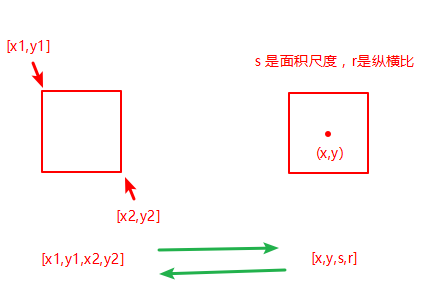源码如下

import numpy as np
#  将[x1,y1,x2,y2]转化成[x,y,s,r]
def convert_bbox_to_z(bbox):
w = bbox - bbox
h = bbox - bbox
x = bbox + w / 2.
y = bbox + w / 2.
s = w * h
r = w / float(h)
return np.array([x,y,s,r]).reshape((4,1))  # 返回四行一列,列向量

# 将[x,y,s,r]转化成[x1,y1,x2,y2]
def convert_x_to_bbox(x,score = None):
'''
:param x: [x,y,s,r]
:param score:  当前候选框的置信度
:return:
'''
w = np.sqrt(x* x)
h = x / w
x1 = x - w /2.
y1 = x - h / 2.
x2 = x + w / 2.
y2 = x + h /2.
if score is None:
return np.array([x1,y1,x2,y2]).reshape((1,4))  # 返回行向量
else:
return np.array([x1,y1,x2,y2,score]).reshape((1,5)) # 返回行向量，带置信度

展开全文• 缩小一个矩形后的坐标点 在一个坐标系中给出一个矩形的四个定点坐标，求将这个矩形缩小一定距离后的坐标矩形要求：四个定点按顺时针或者逆时针排列P0，P1，P2，P3。 具体操作步骤 1、旋转 以P0为旋转，以P0指向...

# 缩小一个矩形后的坐标点

在一个坐标系中给出一个矩形的四个顶点坐标，求将这个矩形缩小一定范围后的坐标。
矩形要求：四个定点按顺时针或者逆时针排列P0，P1，P2，P3。

## 具体操作步骤

### 1、旋转

以P0为旋转点，以P0指向P1向量的角度为旋转角度旋转，角度为atan((P1.y-P0.y),(P1.x-P0.x))
注：逆时针旋转乘上正角度，顺时针旋转乘上负角度值。
旋转后的点计做：R_P0,R_P1,R_P2,R_P3.

### 2、计算旋转后缩小后的坐标

根据P0指向P2向量角度的正负分两种情况计算。在R_P0,R_P1,R_P2,R_P3的坐标值直接相加减即可。

### 3、反向旋转

将R_P0,R_P1,R_P2,R_P3反向旋转回来即可。

## 代码实现

void shrinkPolygon(geometry_msgs::Polygon &out, double shrink_meter)
{
const geometry_msgs::Polygon in = out;
geometry_msgs::Polygon rotate_polygon;
rotate_polygon.points.resize(4);
geometry_msgs::Polygon rotate_shrink_polygon;
rotate_shrink_polygon.points.resize(4);

geometry_msgs::Point32 tem_point32;
geometry_msgs::Point32 rotate_point = in.points;
double theta = atan2((in.points.y - in.points.y),(in.points.x - in.points.x))*(-1.0);
for(size_t i = 0;i < 4;i++)
{
tem_point32 = in.points[i];
rotate_polygon.points[i].x = (tem_point32.x-rotate_point.x)*cos(theta) - (tem_point32.y-rotate_point.y)*sin(theta) + rotate_point.x;
rotate_polygon.points[i].y = (tem_point32.x-rotate_point.x)*sin(theta) + (tem_point32.y-rotate_point.y)*cos(theta) + rotate_point.y;
}

double theta_p02 = atan2((rotate_polygon.points.y - rotate_polygon.points.y),(rotate_polygon.points.x - rotate_polygon.points.x));

if(theta_p02>0)
{
rotate_shrink_polygon.points.x = rotate_polygon.points.x + shrink_meter;
rotate_shrink_polygon.points.y = rotate_polygon.points.y + shrink_meter;

rotate_shrink_polygon.points.x = rotate_polygon.points.x - shrink_meter;
rotate_shrink_polygon.points.y = rotate_polygon.points.y + shrink_meter;

rotate_shrink_polygon.points.x = rotate_polygon.points.x - shrink_meter;
rotate_shrink_polygon.points.y = rotate_polygon.points.y - shrink_meter;

rotate_shrink_polygon.points.x = rotate_polygon.points.x + shrink_meter;
rotate_shrink_polygon.points.y = rotate_polygon.points.y - shrink_meter;
}
else
{
rotate_shrink_polygon.points.x = rotate_polygon.points.x + shrink_meter;
rotate_shrink_polygon.points.y = rotate_polygon.points.y - shrink_meter;

rotate_shrink_polygon.points.x = rotate_polygon.points.x - shrink_meter;
rotate_shrink_polygon.points.y = rotate_polygon.points.y - shrink_meter;

rotate_shrink_polygon.points.x = rotate_polygon.points.x - shrink_meter;
rotate_shrink_polygon.points.y = rotate_polygon.points.y + shrink_meter;

rotate_shrink_polygon.points.x = rotate_polygon.points.x + shrink_meter;
rotate_shrink_polygon.points.y = rotate_polygon.points.y + shrink_meter;
}

for (size_t i = 0; i < 4; i++)
{
tem_point32 = rotate_shrink_polygon.points[i];
out.points[i].x = (tem_point32.x-rotate_point.x)*cos(-theta) - (tem_point32.y-rotate_point.y)*sin(-theta) + rotate_point.x;
out.points[i].y = (tem_point32.x-rotate_point.x)*sin(-theta) + (tem_point32.y-rotate_point.y)*cos(-theta) + rotate_point.y;
}
}


out.points包含矩形的四个顶点坐标，shrink_meter为缩小多少米。

展开全文c++ 算法
• 坐标X = (旋转前X - 中心X) * Math.Cos(角度) - (旋转前Y - 中心Y) * Sin(角度) + 中心X 新坐标Y = (旋转前Y - 中心Y) *Math. Cos(角度) + (旋转前X - 中心X) * Sin(角度) + 中心Y 这是别人给的公式 ...
• 已知倾斜矩形中心点center，长w，宽h，倾斜角theta。顶点计算公式为： void getRectVertex(const cv::Point center,float theta,float w,float h,Point& a, Point& b, Point& c, Point& d) { a.x =...深度学习
• 在平面内,已知一个矩形的四个角坐标,将矩形中心点转动一个角度,求旋转后的角坐标. 也就是已知半径,求每个点旋转后的坐标. 把旋转前和旋转后的点加上中心点看成一个等腰三角形就好解决了,不用扇形公式,而是用...
• 面的形心为其几何中心，通常把三边形和四边形看成密度一致的平面薄片，均匀平面薄片的重心也叫做着平面薄片所占的平面图形的形心。 在平面几何中，三角形三顶点的坐标为：三角形的重心（形心）坐标计算公式： ...
• 步骤： 1. 枚举已有三个两两配对的情况 struct node { double x, y; }; node z; for (int j = 0; j < 3;...2. 因为要求是矩形，所以要找出在直角上的那个，可以利用 两直角边 向量相平面 经验分享 c++
• 先将矩形顶点坐标换算到水平时候的坐标，中心点位置不变。为A（Row- width,Col-height）,B(Row+width,Col-height),C(Rol- width,Col+height),D(Rol+width,Col+height) 然后将A,B,C,D点坐标进行一个theta角度的旋转...
• 图像 矩形 定点/鼠标为中心缩放算法图像 矩形 定点/鼠标为中心缩放算法条件，代码 图像 矩形 定点/鼠标为中心缩放算法 程序中 图像 或 矩形， 按照指定坐标不变缩放算法 条件，代码 图像矩形区域： Rect 缩放系数：...算法
• primes 生成质数列表prod 数组元素的乘积rectint 矩形交集区域sort 按升序排列矩阵元素sortrows 按升序排列行std 标准偏差sum 求和trapz 梯形数值积分var 方差del2 离散拉普拉斯diff 差值和微分估计gradient 数值...
• * 求取多边形的中心位置(该方法是最原始版，之前因为用的少，所以没有发觉到逻辑上存在错误)~ * Added By Bruce Yang on 2012.09.02.15.30~ * 实践证明，这个方法的逻辑是错误的，汲取这个教训~ */ +(b2Vec2) ...float
• 已知两点坐标和第三点与两点的距离,怎么计算第三点坐标假设第三那点坐标为(x,y),先求出已知两点坐标的直线方程,再根据点到线的距离算出第三点坐标点到直线距离公式可以不代坐标计算?直线L1有用吗.过(0,2)作L2垂线使...
• 测试没问题，我用的是原始坐标；要注意的是坐标转换问题，要看当前是属于什么坐标系 我国常用的地心系及对应椭球： 为什么CGCS2000和WGS84要略微有些偏差？这是因为WGS84系是GPS的坐标系，而我国北斗定位则...
• python opencv minAreaRect 生成最小外接矩形的方法使用python opencv返回点集cnt的最小外接矩形，所用函数为 cv2.minAreaRect(cnt) ，cnt是集数组或向量(里面存放的是坐标)，并且这个点集不定个数。...
• 现在对矩形B进行旋转，如旋转90度，那C点坐标为多少呢？其实如果C点是属于矩形B的话，旋转B时，C点会自动进行旋转，C点相对D点的坐标是不变的，并不需要我们额外处理，当如果C点不是属于B，但旋...
• ## 求解最小外接矩形

千次阅读 2018-12-27 14:15:43
給出一个平面点集S，求一个面积最小的矩形使其包含S所有的。 预备知识：  在求解这道题之前我们先要了解一些关于凸包的知识。  什么是凸包？简单地说，对于一个平面点集S，我们把完全包含该...
• 最近要做附近的人查询算法，在网上找了相关示例，发现代码有些问题，经过我整理...1.经纬度坐标实例，方便实例化和传值。 /// /// 经纬度坐标 /// public class Degree { /// /// 构造函数 /// /// 经度c# 算法 经纬度
• 1. 首先图像的左上角为原点建立坐标系，往右往下为正向，围绕图像的中心位置进行旋转 逆时针90度：x=2*(x/w-0.5) ,y=2*(y/h-0.5) , X=y, y=-x, x=(x+1)/2*h,y=(y+1)/2*w 顺时针90度：x=2*(x/w-0.5) ,y=2*(y/h-...
• 开发中碰到一个坐标转换的算法问题，终于解决了，在此分享下。 java代码实现如下： private static Point calcNewPoint(Point p, Point pCenter, float angle) { // calc arc float l = (float) ((angle *...Rotate
• 给定一个非重叠轴对齐矩形的列表 rects，写一个函数 pick 随机均匀地选取矩形覆盖的空间中的整数。 提示： 整数是具有整数坐标矩形周边上的包含在矩形覆盖的空间中。 第 i 个矩形 rects [i] = [x1，y1...
• ## 多边形重心和中心

千次阅读 2020-10-02 07:06:12
目录 ...获取多边形外接矩形，获取最大最小XY（也就是外接矩形的四个角坐标：Xmax、Xmin、Ymax、Ymin） static double XMax, XMin, YMax, YMin; double CenterX = (XMax + XMin) / 2; dou.
• 坐标其实很简单，但...目 录—、极坐标的基本概念二、极坐标和直角坐标的转换三、直角坐标系下的曲线方程转换为极坐标系下的曲线方程 (1）圆心在原点的圆: (2）圆心过原点的椭圆方程: (3）直线四、对称中心不在原...
• 图4（a）显示了center pooling的操作：首先由骨干网络得到一个特征图，同时判断该特征图中是否存在一个中心点，然后需要找到该点水平方向及垂直方向上的最大值，并将其进行相加。通过这样做，center pooling有助于更...
• ## 旋转矩形

千次阅读 2018-12-14 00:45:21
角，得到v’，假设 v坐标是(x, y) ，那么可以推导得到 v’坐标（x’, y’)(设原点到v的距离是r，原点到v的向量与x轴的夹角是 ϕ  )  x = rcosϕy = rsinϕ   x ′= rcos ( θ + ϕ ) y ′= rsin ( ...
• 多个角度扫描同一个物体时，通常会在物体上或者其他固定支架上...在三维重建之前，需要在二维图像上提取标志点中心坐标，本文讲解一种利用梯度提取粗轮廓，然后进行亚像素获取，连接轮廓，椭圆拟合得到中心点的方法。c++ 计算机视觉 算法
• 因此，当您对极坐标的常规网格进行采样时，这些坐标在转换为矩形时将形成圆形形状 大多数都集中在cricle的中心，当我们从中心移动到外部区域时，之间的距离增加。 %regular grid generated for r and t [THETA ......